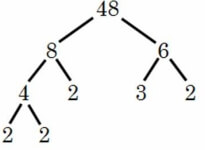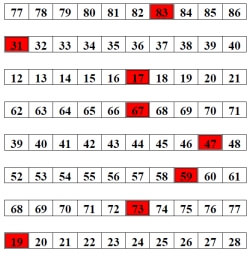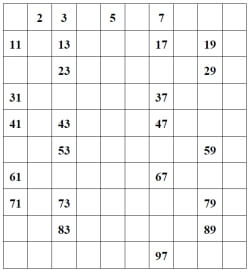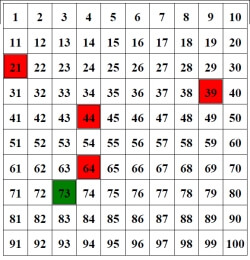Worksheets and No Prep Teaching Resources
Math Worksheets

# Factor Worksheets

Understanding factoring can affect student success in math, and these unique worksheets will help students almost effortlessly master prime numbers, composite numbers, and number theory. Polynomial classification, as well as adding, subtracting, multiplying and dividing worksheets, polishes these advanced skills for older students in creative ways. Valuable number theory and polynomial review printables make sure students won't forget these essential math concepts.Prime NumbersFill-in all of the prime numbers up to 100 Color in the prime numbers up to 100 (5 are given) Color in the prime numbers up to 100 (2 are given) Rows - Color in the prime numbers (1 given in each row) Rows - Color in the prime numbers

Composite NumbersFill-in all of the prime numbers up to 100 Color in the prime numbers up to 100 (5 are given) Color in the prime numbers up to 100 (2 are given) Rows - Color in the prime numbers (1 given in each row) Rows - Color in the prime numbers

Prime or Composite NumbersColor in the prime numbers up to 100 (5 are given) Color in the prime numbers up to 100 (2 are given) Rows - Color in the prime numbers (1 given in each row) Rows - Color in the prime numbers

Number Theory
Prime or composite
Divisibility: complete the table
Divisibility: fill in the missing digit
List all of the factors
Prime factorization
Greatest common factor
Least common multiple
Open-ended number theory questions
Number theory review printable

Polynomial Worksheets
Classify as a monomial, binomial, or trinomial
Subtracting Polynomials
Multiplication (Monomial by Polynomial)
Multiplication using FOIL (Binomial by Binomial)
Multiplication (Polynomial by Polynomial)
Dividing a Monomial by a Monomial
Dividing a Polynomial by a Monomial
Mixing Addition, Multiplication, and Division in one problem.
A mix of all the different operation worksheets.
Common Factors: Factor out the GCF
Common Factors: Write as a Product of Two Binomials
Factoring: Perfect-Square Trinomials
Factoring: Perfect-Squares (more difficult)